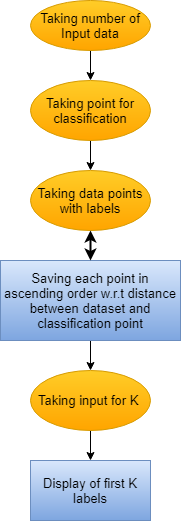# KNN ALGORITHM USING LINKED LIST

Nearest Neighbours algorithm has been in action for the last sixty years. It is mainly used in statistical estimation and pattern recognition, as a non-parametric method, for regression and classification. The main aim of the K-Nearest Neighbor algorithm is to classify a new data point by comparing it to all previously seen data points. The classification of the k most similar previous cases are used for predicting the classification of the current data point. It is a simple algorithm which stores all available cases and classifies new cases based on a similarity measure (e.g., distance functions).
This algorithm suggests that if you’re similar to your neighbours, then you are one of them.
Whenever a prediction is required for an unseen data instance, it searches through the entire training dataset for k-most similar instances and the data with the most similar instance is finally returned as the prediction.

## Methodology

• At first the user is requested to enter number of datapoints in his dataset and then asked about the point to be classified.
• The user enters all the data points with their respective labels.
• The distance between the point to be classified and the points in dataset is calculated and are arrange in ascending order of distance between them.
• The value of K is asked from the user and the first k labels are shown as the output.

## FlowchartView Github